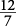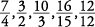## Proper and Improper Fractions

A fraction like, where the numerator is smaller than the denominator, is less than one. This kind of fraction is called a proper fraction. But sometimes a fraction may be more than one or equal to one. This is when the numerator is larger than the denominator or equal to the denominator. Thus,is more than one andis equal to one. These kinds of fractions are called improper fractions.

• Examples of proper fractions:• Examples of improper fractions: